American Journal of Applied Mathematics
Volume 3, Issue 4, August 2015, Pages: 169-173

Trisection of Any Angle and Consequentially the Division of Any Angle Into Any Number of Equal Parts

Lorna A. Willis

Department of Physics, Faculty of Science and Technology, University of the West Indies, Mona Campus, Jamaica, West Indies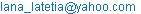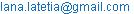Lorna A. Willis. Trisection of Any Angle and Consequentially the Division of Any Angle Into Any Number of Equal Parts. American Journal of Applied Mathematics. Vol. 3, No. 4, 2015, pp. 169-173. doi: 10.11648/j.ajam.20150304.11

Abstract: Historically, a contrived trisected line was used to trisect any other line, using the principle of projection. This is in essence about relationship and its accomplishment is about working backwards. Loosely speaking, any angle comprises two connecting lines. Attempts at trisecting any angle, which is dividing it into three equal parts, failed. In this paper any angle is defined as a unique pair of arc and chord of sector of a circle irrespective of arc radius. Two theorems viz. Equal arcs have equal central angles and equal chords have equal central angles are combined to establish a unique relationship between a pair of arc-chord and its composite of three identical pairs of arc-chord, thereby revealing a cyclic trapezium, where the base defines the angle, and each equal edge defines each of the equal trisected parts of this angle. For a range of angles between 0o and 360o, this relationship is expressed as Lorna Graph, which becomes the practical tool for trisection of any angle, using the working backwards approach. This approach is extended to division of any angle into any number of equal parts.

Keywords: Cyclic Trapezium, Working Backwards, Practical Tool

1. Introduction

History tells us that one of the earliest geometric constructions was about the bisection of an angle, which is the division of angle into two equal parts.

Mathematicians sought to take this achievement to the next level by considering the trisection of any angle, which is dividing it into three equal parts. Those efforts failed, and it was – "Not until the 19th Century was that proved to be impossible."

The trisection of a line, any line, was at some time not possible, at least directly, since the length of each equal part was not, nor could be, known exactly, with the use of only a straight edge. However, mathematicians have succeeded in trisecting any line by using a known trisected line and determining its projections on the line to be trisected, then working backwards succeeded in trisecting the line.

By mimicking this approach it will be demonstrated that the trisection of any angle is possible by considering the following;

1   A line is defined by one parameter, its length;

2   An angle, a circular measure, is defined by a unique pair of arc and associated chord of a sector of a circle irrespective of arc radius;

3   An angle and its trisected parts would need to be characterized by one length for the angle (composite), and three equal lengths for the three equal angles.

2. Definition, Relationships, Graph and Deductions

The word trisection refers to division into three equal parts. Trisection of a line has not been possible by direct action, but by the use of a projection of a known contrived trisected line, trisection of any line has been made possible.

Analogously, trisection of any angle is its division into three equal angles. "Not until the 19th Century that was proved to be impossible". However, what is impossible by one way of thinking becomes possible by another way of thinking.

According to the Oxford Mathematics Study Dictionary, by  Frank Tapson, p. 12, "An angle is made when two straight lines cross or meet each other at a point, and its size is measured by the amount one line has been turned in relation to the other". Many educators have defined an angle as "that which is made by two connecting half rays". The projection of one line on another is in essence about relationship, and its accomplishment is about working backwards. In this paper a different approach is used to define any angle as a unique pair of arc and chord of a sector of a circle irrespective of arc radius.

Two theorems of relevance to this paper are:- Equal arcs have equal central angles and Equal chords have equal central angles. By combining these two theorems we have identical or equal pairs of arc and chord have equal central angles.

Three equal pairs of arc and chord define the trisection of any angle. This leads to the identification of a cyclic trapezium as the contrived known form comprising four lengths of line elements which define the angle and its three trisected parts. The relationship between the base and any of the equal edges, for the range 0o to 360o, is a Lorna graph; which is the practical tool for working backwards to trisect any angle.

Differently contrived forms of m sides, likewise, accomplish the task of dividing any angle into (m-1) equal parts.

In this paper any angle, which is a circular measure is defined as a unique pair of arc and chord of a sector of a circle irrespective of arc radius. This is illustrated in Figure 1 which shows three different arc radii, viz r , 2r, 3r.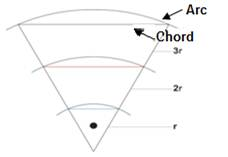Figure 1. Angle defined by pair of arc and chord

Any angle which is trisected will be defined by

1   A unique pair of arc and chord- Figure 1.

2   Three identical pairs of arcs and chords that define each of the three equal angles comprising the whole angle; as is illustrated in Figure 2.  which reveals three cyclic trapezia.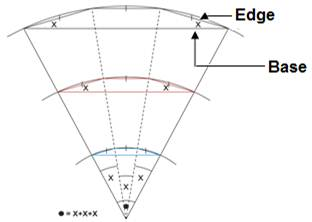Figure 2. Cyclic Trapezium defining trisection irrespective of arc radius

Consider a straight angle or 180o as is illustrated in Figure 3; which effectively demonstrates that the base angle in the cyclic trapezium equals one of the angles of trisection. This relationship applies to any cyclic trapezium which defines trisection, since an equivalent problem is described.

Hence, the details in Figure 2.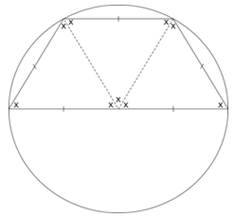Figure 3. Relationship between base angle of cyclic trapezium and each angle of trisection

The cyclic trapezium appears ‘differently’ with respect to kind of angle (composite) as is illustrated in Figure 4.

For an acute angleFigure 4. Relationship between acute composite angle and each angle of trisection

For an obtuse angle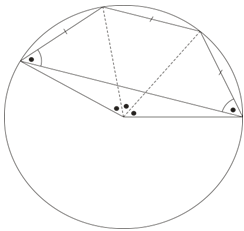Figure 5. Relationship between obtuse angle and each angle of trisection

For a reflex angle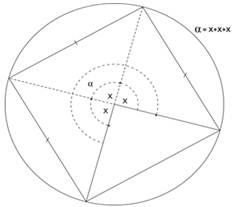Figure 6. Relationship between reflex angle and each angle of trisection

For 360o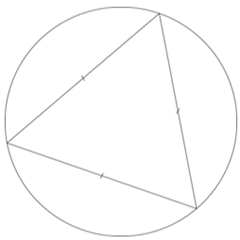Figure 7. Relationship between 360o and each angle of trisection

This equilateral triangle is a special case of a cyclic trapezium, in which the major chord, its base, vanishes, i.e. its magnitude is zero.

In Figure 2 the sameness of the base angles for all three trapezia demonstrates a unique relationship between minor chord and major chord. For a range of angles between 0o and 360o this unique relationship can be expressed as a Lorna graph, which in essence is a quantification of the relationship.

Generation of the Lorna graph

a)  Construct a line segment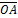, arbitrarily chosen, whose magnitude is the arc radius r, as shown;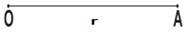Figure 8. Line segment of arc radius r

b)  With radius r, construct an arc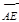as shown;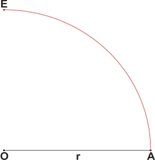Figure 9. Arc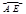c)  With new radius much less than r, arbitrarily chosen, construct three identical minor arcs viz.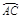,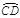and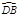, as shown;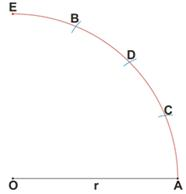Figure 10. Three identical minor arcs

d)  With this radius, identify mi, the minor chord on the grid as shown in Figure 11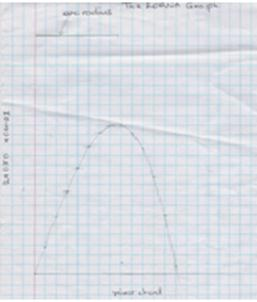Figure 11. Identification of minor chord

e)  Connect O to B as shown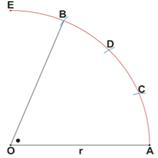Figure 12. Line OB

f)   Connect A to B to obtain major chord mj,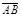as shown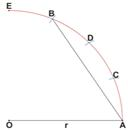Figure 13. Major chord

g)  Obtain compass extent of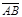and use it to identify mj the major chord on grid as shown in Figure 11.

h)  Repeat  d) to g) for varying magnitudes of mi

i)   Note, the number of points generated for the Lorna Graph depends on the number of values of mi

As the straight edge chosen is flexible this flexibility is used to complete drawing of graph in Figure 11.

2.1. The Effect of Arc Radius on the Graph

As the magnitude of the arc radius decreases the size- not the form- of the graph shrinks.

As the magnitude of the arc radius increases, the size of the graph increases.

2.2. Using the Graph- the Practical Tool for Solving the Angle Trisection Problem

Recognize any angle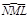as shown defined by arc radius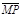= r, the magnitude of arc radius of Graph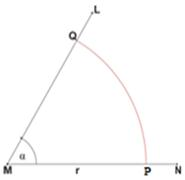Figure 14. AngleWith compass extent determine mj =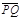as shown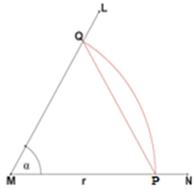Figure 15. mj =With this compass extent identify from the graph the value of mi

With compass extent equal to mi construct arcs at R and S as shown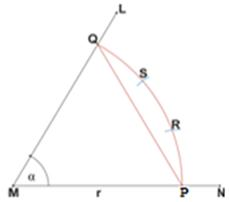Figure 16. Construction of arcs at R and S

Construct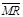,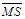to complete trisection as shown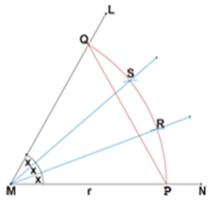Figure 17. Construction ofandFrom the foregoing it can be deduced that similarly any angle can be divided into any number of equal parts by reference to the table shown.

Table 1. Shapes in relation to number of edges and number of angles

 Shape Number of equal edges Number of equal angles Cyclic Pentagon 4 4 Cyclic Hexagon 5 5 Cyclic Heptagon 6 6 Cyclic Octagon 7 7 Cyclic Nonagon 8 8 Cyclic Decagon 9 9It is then a matter of constructing the graph that relates the base of the relevant shape to anyone of its equal edges and working backwards.

3. Conclusions

It is useful to note the following;

By refining the historical definition of an angle it became possible to use two established theorems in the solution of the trisection problem;

Borrowing ideas from one situation for use in another is a beneficial exercise;

The combination of the two referred theorems allowed a correlation between two length parameters, the major chord and the minor chord;

The referred correlation is quantified as a graph which is the practical tool for working backwards to facilitate  the trisection process;

This entire process leads to differently contrived forms of   m sides for accomplishing the task of dividing any angle into (m-1) equal parts.

4. Closure

Trisection of any angle is its division into three equal angles. "Not until the 19th Century was that proved to be impossible". Using a historically different approach, an angle is defined by a unique pair of arc and corresponding chord not limited by specific arc radius.

A combination of two established theorems which relate equal arcs and equal chords to their central angles revealed a specific shape, the cyclic trapezium, which relates a composite angle to each of its trisected angles.

For a range of 0o to 360o, this relationship between base and any equal edge results in a Lorna graph which is the practical tool for working backwards to trisect any angle. By extension, shapes of insides, (m-1) being equal, accomplish the task of dividing any angle into (m-1) equal angles.

References

2. Tapson, Frank, "Oxford Mathematics Study Dictionary", p.46, Oxford University Press, 2006
3. Claphan, Christopher, "The concise Oxford Dictionary of Mathematics Oxford Reference", p. 184 Oxford University Press, New York, 1990
4. Edited by Iyanaga, Shokichi; Kawada, Yukiyosi (translated by Mathematical Society of Japan, American Mathematical Society) "Encyclopedic Dictionary of Mathematics: Mathematical Society of Japan" p. 588 mit press, Cambridge 1968
9. Encyclopedia International, Grolier Incorporated, New York/Montreal/Mexico City/Sydney 1965,1964, 1963 p. 229
10. Encyclopedia Britannica Inc. 15th Edition, p.130. William Benton Publisher 1943-1973; Helen Hemingway Benton Publisher 1973-1974 Founded 1968, © 1978
11. Penney, David E "Perspectives in Mathematics", p.18 The University of Georgia, W.A Benjamin Inc. Menlo Park, California ©1972
12. Robinson, Gilbert D.B, Collier’s Encyclopedia with Bibliographical Index Vol 10/24; p. 688Maxwell Macmillan International Publishing Group Canada 1992
13. Bennett, Albert B Jr, Nelson, l. Ted "Mathematics of Elementary Teacher: A Conceptual Approach" 5th Edition p.722. Mc Graw Hill Higher Education isbn 0-07-23481-7 © 2001
14. New Encyclopedia Britannica, "Macropedia-Knowledge in Depth" Vol 19 Founded 1768 15th Edition, Inc. p.890 Jacob E. Safra, Chairman of the board Jorge Aguillar-Cuiz, President. London/New Delhi/Paris/Seoul/Sydney/Taipei/Tokyo © 2005
15. Gardner, Martin "Entertaining Science: Experiments with everyday", illustrated by  Anthony Ravielli (n.d, publisher not given)
16. J.f.s., Chamber’s  Encyclopedia New Revised Edition Vol 13 p.791Spain-Turing International Learning Systems Corporation Limited, London © 1966 Pergamon Press

 Contents 1. 2. 2.1. 2.2. 3. 4.
Article ToolsAbstractPDF(300K)